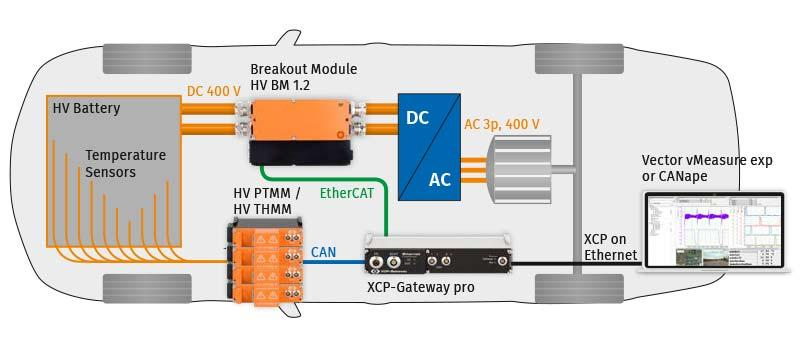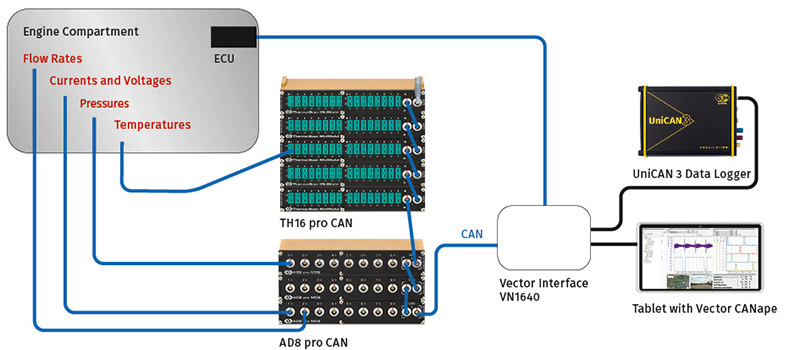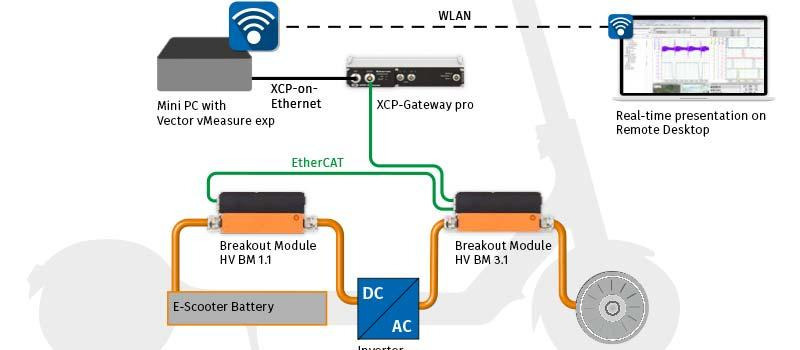Skip to main content
Use Cases# Use Cases

We support our customers with innovative measurement solutions for product development. Our measurement technology is used in many areas and at all stages of development. Especially in the area of high-voltage safe measurement technology, we were able to develop new solutions together with our customers. In the following we present a selection of possible applications of CSM measurement systems.

## High-Voltage Temperature Measurement

Temperatures are an important factor for the performance of high-voltage batteries, inverters and electric motors. Here you can find out how temperatures are measured in a high-voltage safe way.## High-Voltage Current and Voltage Measurement

Precise current and voltage measurements are the basis for extensive analyses in the electric power train. Here we have put together various solutions for you.## High-Voltage Power Measurement

Power measurement and the analysis of power parameters (e.g. active power, apparent power, blind power) are important steps in the development of powertrain components. These examples show how such measurements are performed.## High-Voltage Measurement of Analog Voltages

Numerous measured variables that can be measured with standard sensors must also be acquired in the high-voltage environment. Find out here how, for example, accelerations, pressures, moisture, can be reliably measured.## Temperature Measurement

Temperatures must be measured at many points in the vehicle. In these applications you will learn how temperatures can be measured easily and precisely.## Current and Voltage Measurement

Currents and voltages must also be measured in low voltage environments. Here we show you how such measurements are carried out with CSM measurement technology.## Measurement of Analog Voltages

Many variables are measured with sensors that output the respective measured variable (e.g. acceleration, moisture, pressure) as analog signals. Here you will find examples of such measurements.## Measurement of Mechanical Stress

Here you will find our application examples on the performance of structural and fatigue measurements and mechanical load with strain gauges.